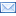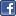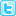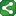## Monday, March 2, 2009

### How to calculate number of years and number of days between 2 dates;

How to calculate number of years and number of days between 2 dates;

Exploring the yrdif and datdif functions in SAS as well as INTCK function:
There are several ways to calculate the number of years between two dates and out of all the methods, YRDIF function results the most accurate value.

Syntax:

ageinyrs = YRDIF(birthdate, enddate, 'act/act');
ag_indays = DATDIF(birthdate, enddate, 'act/act');

act/act” will gives us the actual interval between the two dates.

The YRDIF function returns the calculated years between two SAS date values. The returned value will be a precise calculation, including multiple decimal places. Whereas with INTCK function will just give the rounded value like 10, 11 and not like 10.2 and 11.5.

Syntax:
Using YRDIF function:
To know the interval between two dates in Years:

data _null_;
sdate="12mar1998"d;
edate="12jun2008"d;
years=yrdif(sdate,edate,'act/act');

put years;
run;

Output: 10.2535 yrs

Using DTDIF function:
To know the interval between two dates in Days:

data _null_;
sdate="12mar1998"d;
edate="12jun2008"d;
days=datdif(sdate,edate,'act/act');

put days;
run;

output: 3745 days

Using the INTCK function:

data _null_;
sdate="12mar1998"d;
edate="12jun2008"d;
years=intck('year',sdate,edate);
put years;

run;

output:10 years

The calculation for the number of years from INTCK function is different from that generated by YRDIF. This is because the INTCK function bases the interval from the start of the respective intervals.

The INTCK function returns the integer count of the number of intervals in years, months or days between two dates.

Ex:
To know the interval between 2 dates in days:

data _null_;
sdate="12mar1998"d;
edate="12jun2008"d;
days=intck(‘days’,sdate,edate);

put days;
run;

result: 3745 days

To know the interval between 2 dates in months:

data _null_;
sdate="12mar1998"d;edate="12jun2008"d;
months=intck('months',sdate,edate);

put months;
run;

result: 123 monthsAnonymous said...

Thanks!Anonymous said...

how do you include all the days except sundays??ShareThis